Get instant live expert help with Excel or Google Sheets“My Excelchat expert helped me in less than 20 minutes, saving me what would have been 5 hours of work!”

#### Post your problem and you’ll get Expert help in seconds.

Your message must be at least 40 characters
Our professional Expert are available now. Your privacy is guaranteed.

# Examples of VLOOKUP with the IF Condition

The VLOOKUP function is a powerful one. When you combine it with the IF function, it can create a more dynamic formula

## Final Formula

`=VLOOKUP(7,A3:C27,IF(J3="Sales Rep",2,3),FALSE)`

##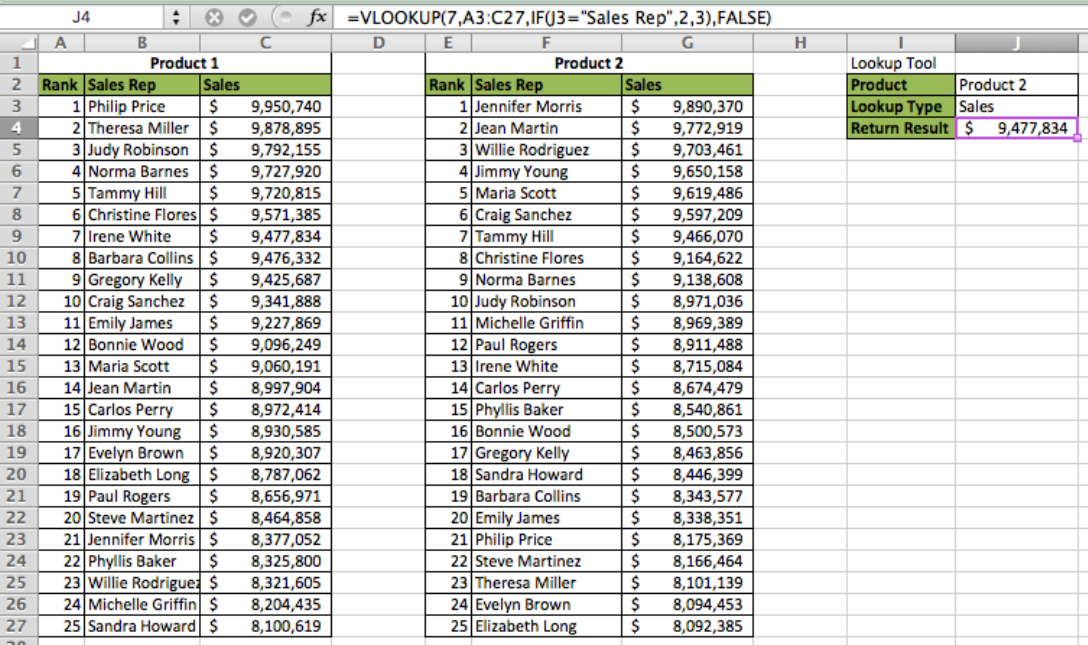Figure 1 – Final result

## Setting up the Data

First, we will setup our data so that the Lookup Array is Column A, the Return Arrays are Column B and Column C. Next, we’ll create a second table where Column E is the Lookup Array and the Return Arrays are Column F and Column G. Then, we will use cells I2:J4 as our Lookup tool where we will enter our formulas. Be sure to label the tables “Product 1” and “Product 2” in cells A1 and E1 respectively. We will reference these labels later in the tutorial

##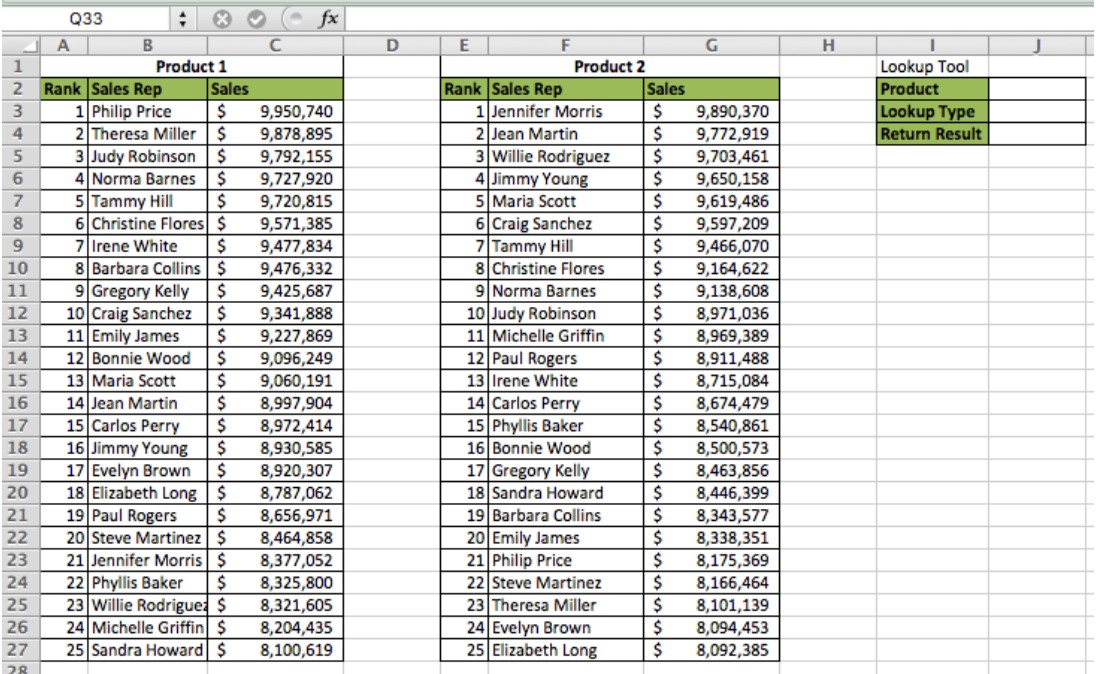Figure 2 – Setting up the Data

Now that that we have the data setup we can walk through the formulas

## Applying the IF formula Formula

The IF formula can be used to test for logic. If the specified logic test returns TRUE, then Excel will return a specified value. If FALSE, it will return a different specified value

IF Syntax:

`=IF(Test Logic, Value if TRUE, Value if FALSE)`

• In cell J2, enter this formula =IF(C3>G3,A1,E1)

##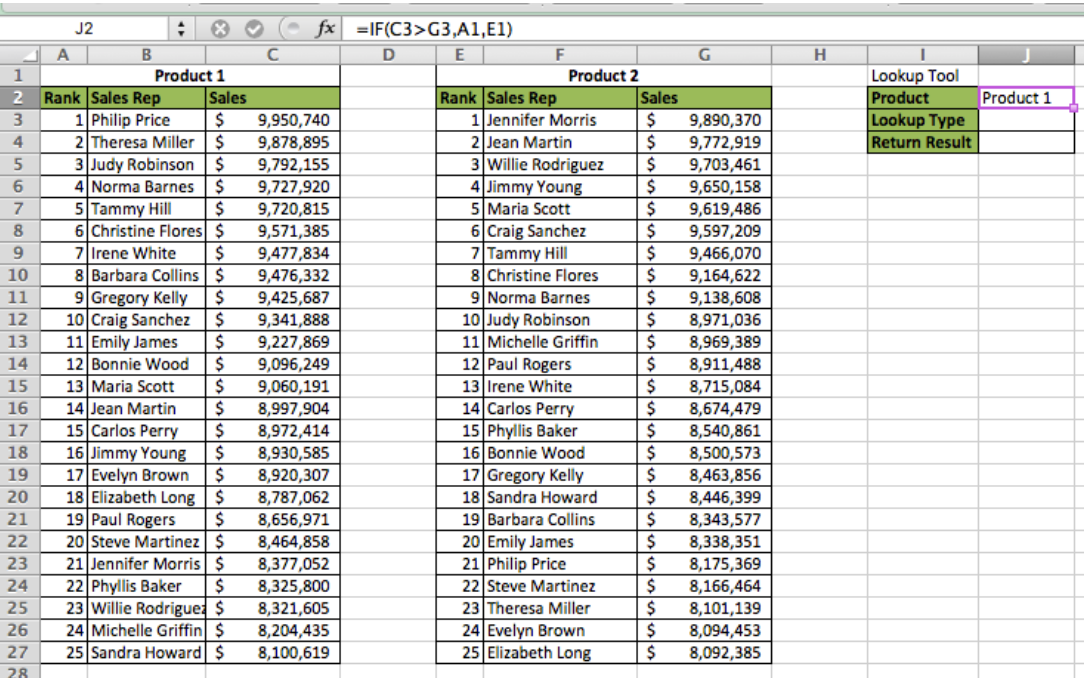Figure 3 – Applying the IF Formula

We can see in this example how the formula returns “Product 1” because C3 is greater than G3. If this wasn’t the case, the formula would have returned “Product 2”

## Applying the VLOOKUP Formula

The VLOOKUP function allows us to lookup a specified value within a column of data and then return a value adjacent to the matching value

VLOOKUP Syntax:

`=LOOKUP(Lookup Value, Table Array, Return Column, Approximate Match [TRUE/FALSE] `

• Type the number 7 in cell J3
• In cell J4, type this formula =VLOOKUP(J3,A3:C27,2,FALSE)

##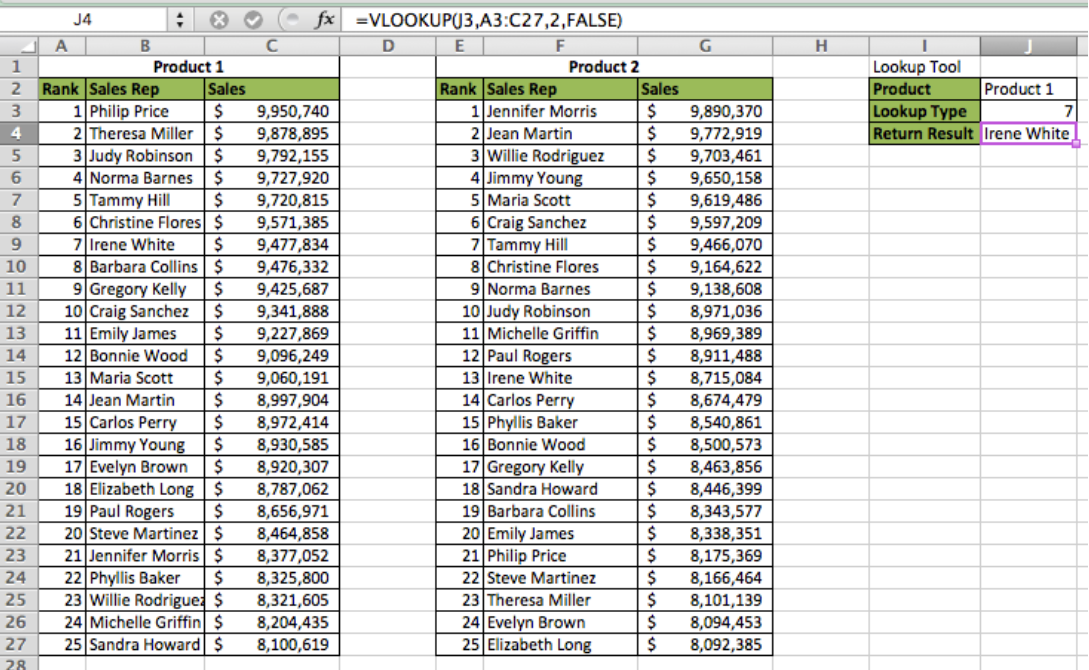Figure 4 – Applying the VLOOKUP Formula

We notice in this Example how the formula returns “Irene White because she has the 7th top ranking sales since we specified “7” for the Lookup Value to find in the Table Array

## Nesting VLOOKUP Inside IF Function

There are two Main ways to nest the IF and VLOOKUP functions. We can either nest VLOOKUP within the IF function to change Table Arrays dynamically, or we can nest IF within the VLOOKUP function to change the variables within the VLOOKUP function First we will look at nesting the VLOOKUP inside of an IF function

IF (VLOOKUP) Syntax:

`=IF(Test Logic,VLOOKUP 1, VLOOKUP 2)`

• Delete the data in cell J4
• In cell J4 type this formula `=IF(J2="Product 1",VLOOKUP(J3,A3:C27,2,FALSE),VLOOKUP(J3,E3:G27,2,FALSE))`

##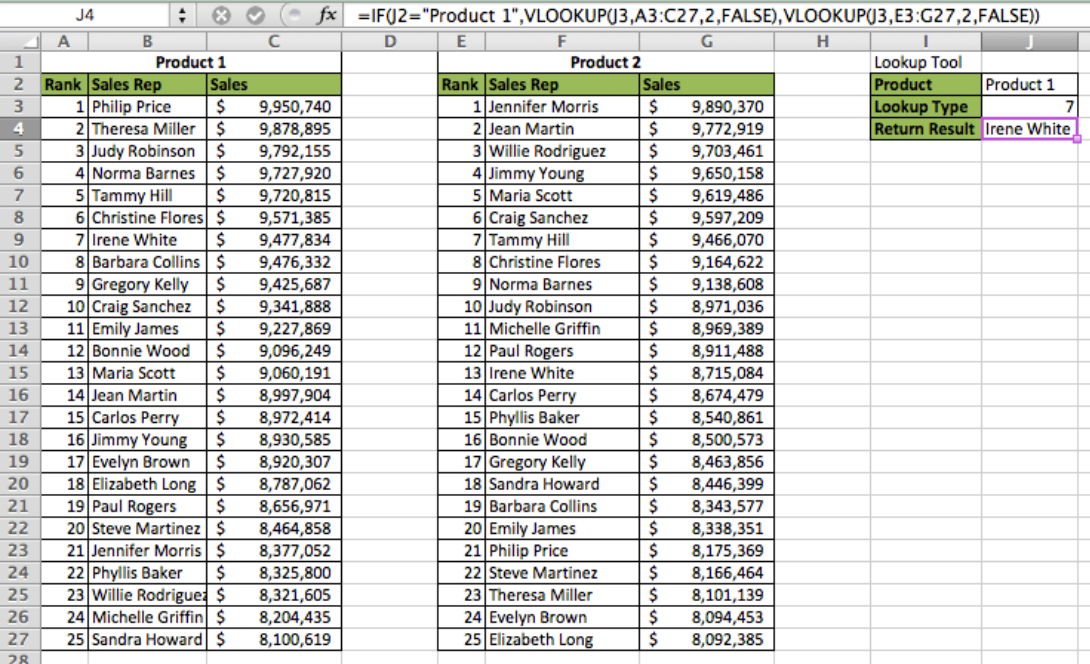Figure 5 – Nesting VLOOKUP inside of IF Function – Product 1

We notice in this Example that the result is “Irene White” because she has the 7th rank sales and is located under the Product 1 table. If we type “Product 2” in cell J2, the result becomes “Tammy Hill”

##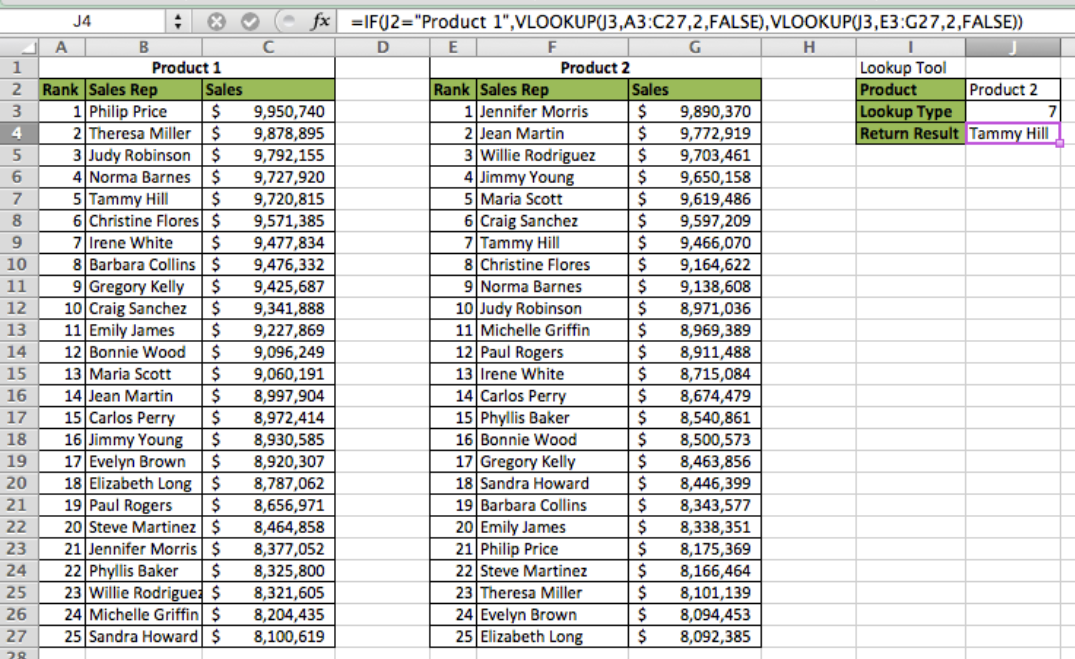Figure 6 – Nesting VLOOKUP inside of IF Function – Product 2

## Nesting IF Inside VLOOKUP Function

Now we can look at nesting the IF function within the VLOOKUP formula

VLOOKUP(IF) Syntax:

`=VLOOKUP(Lookup Value,Table Array,IF(Test Logic, Return Column 1, Return Column 2, Approximate Match [TRUE/FALSE])`

• Delete the data in cell J3
• Type “Sales” in cell J3
• In cell J4, type this formula `=VLOOKUP(7,A3:C27,IF(J3="Sales Rep",2,3),FALSE)`

##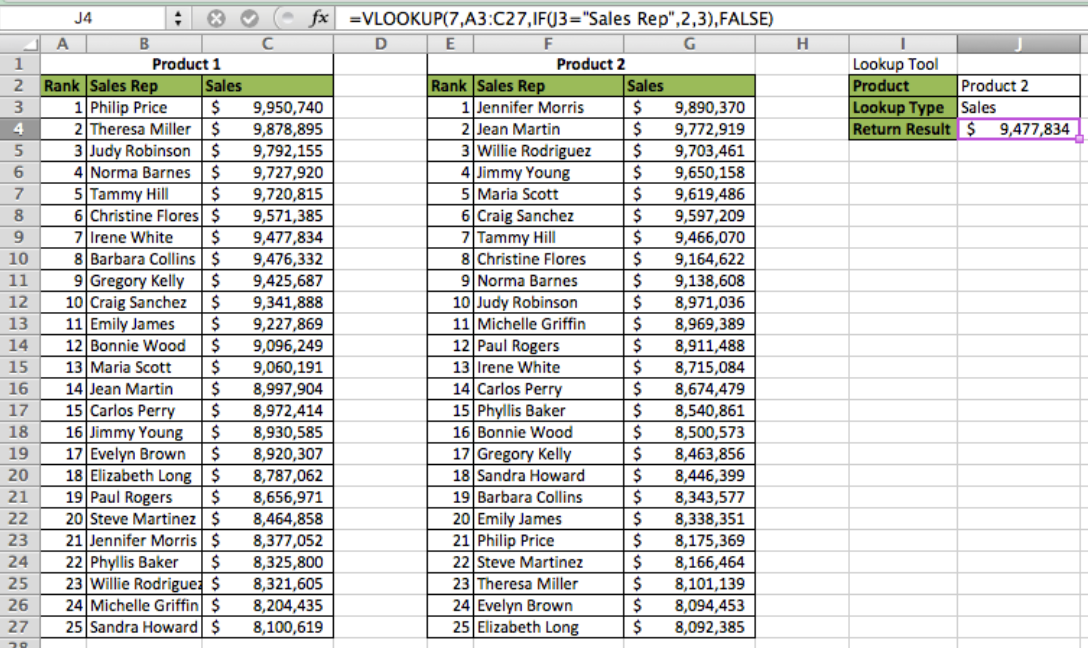Figure 7 – Nesting VLOOKUP inside of IF Function – Product 2

We notice in this example the formula looked at the Sales column because we specified it in the IF function. We then hard coded “7” as the lookup value. Therefore, the formula returned the sales for the 7th ranked sales rep. If we entered “Sales Rep” in J3, the formula would have returned “Irene White”

Most of the time, the problem you will need to solve will be more complex than a simple application of a formula or function. If you want to save hours of research and frustration, try our live Excelchat service! Our Excel Experts are available 24/7 to answer any Excel question you may have. We guarantee a connection within 30 seconds and a customized solution within 20 minutes.

Are you still looking for help with the VLOOKUP function? View our comprehensive round-up of VLOOKUP function tutorials here.

### Did this post not answer your question? Get a solution from connecting with the expert.Another blog reader asked this question today on Excelchat:
Solution examplesI filtered data in column A (it is labeled ID #) of a data set. On my next worksheet, column A is also the ID #, but it is a different data set. I want to filter the ID #'s the same for the two sheets, but how do I do that being that I am working with two different sets of data?
Solved by V. H. in 22 minsCould you help me by explaining the IF function?
Solved by G. L. in 24 minsVLOOKUP help. Trying to input data from database into new table
Solved by S. Q. in 40 minsI'm looking for a formula or format for if there is data in c4 on a sheet... It adds extra information underneath
Solved by X. E. in 20 minshi, my vlookup wont work in some rows but does in others. its temperemental. e.g it will work on rows 10 to 15, then not row 16 and 17, but the will for the rest.
Solved by K. Q. in 21 mins## Subscribe to Excelchat.coAnother blog reader asked this question today on Excelchat: